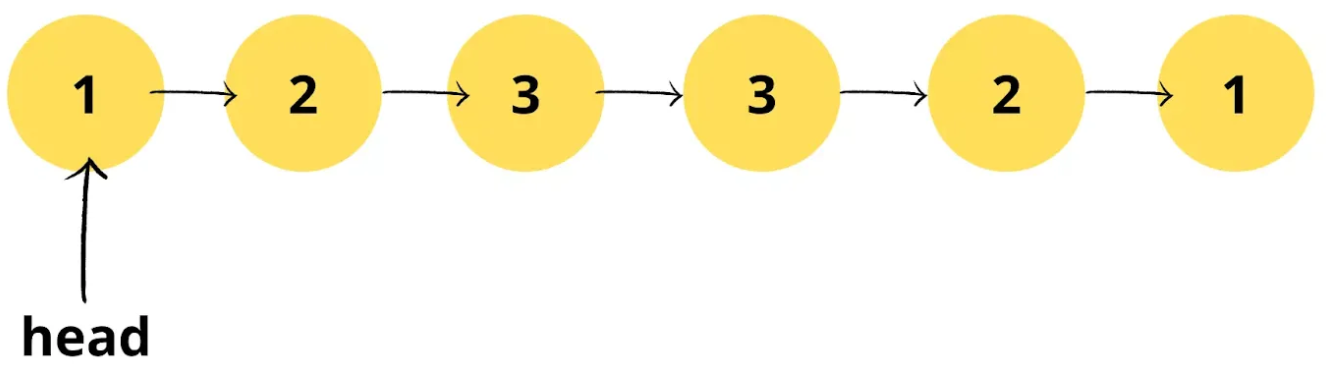# Check if given Linked List is Plaindrome

Check if the given Linked List is Palindrome

Problem Statement: Given the head of a singly linked list, return true if it is a palindrome.

Examples:

```Example 1:
Output:
true
Explanation: If we read elements from left to right, we get [1,2,3,3,2,1]. When we read elements from right to left, we get [1,2,3,3,2,1]. Both ways list remains same and hence, the given linked list is palindrome.```
```Example 2:
Input:
Output:
false
Explanation: When we read elements from left to right, we get [1,2]. Reading from right to left, we get a list as [2,1]. Both are different and hence, the given linked list is not palindrome.```

Solution: Using the extra data structure

Approach:

We can store elements in an array. Then check if the given array is a palindrome. How to check if an array is a palindrome?

Let’s take a string, say “level” which is a palindrome. Let’s observe a thing.

So we can see that each index letter is the same as (length-each index -1) letter.

The same logic required to check an array is a palindrome.

Following are the steps to this approach.

• Iterate through the given list to store it in an array.
• Iterate through the array.
• For each index in range of n/2 where n is the size of the array
• Check if the number in it is the same as the number in the n-index-1 of the array.

Code:

## C++ Code

``````#include<bits/stdc++.h>
using namespace std;

class node {
public:
int num;
node* next;
node(int val) {
num = val;
next = NULL;
}
};

node* newNode = new node(val);
return;
}

while(temp->next != NULL) temp = temp->next;

temp->next = newNode;
return;
}

vector<int> arr;
}
for(int i=0;i<arr.size()/2;i++)
if(arr[i] != arr[arr.size()-i-1]) return false;
return true;
}

int main() {
return 0;
}
``````

Output: True

Time Complexity: O(N)

Reason: Iterating through the list to store elements in the array.

Space Complexity: O(N)

Reason: Using an array to store list elements for further computations.

## Java Code

``````import java.util.*;
class Node {
int num;
Node next;
Node(int val) {
num = val;
next = null;
}
}
class TUF{
static Node insertNode(Node head,int val) {
Node newNode = new Node(val);
}

while(temp.next != null) temp = temp.next;

temp.next = newNode;
}

ArrayList<Integer> arr=new ArrayList<>();
}
for(int i=0;i<arr.size()/2;i++)
if(arr.get(i) != arr.get(arr.size()-i-1)) return false;
return true;
}

public static void main(String args[]) {
System.out.println("True");
else
System.out.println("False");

}
}``````

Output: True

Time Complexity: O(N)

Reason: Iterating through the list to store elements in the array.

Space Complexity: O(N)

Reason: Using an array to store list elements for further computations.

## Python Code

``````class Node:
def __init__(self, val):
self.val = val
self.next = None

# utility function to insert node at the end of the linked list
newNode = Node(val)
while temp.next != None:
temp = temp.next
temp.next = newNode

# utility function to check if the linked list is palindrome or not
arr = []
for i in range(len(arr)//2):
if arr[i] != arr[len(arr)-i-1]:
return False
return True

if __name__ == "__main__":

Output: True

Time Complexity: O(N)

Reason: Iterating through the list to store elements in the array.

Space Complexity: O(N)

Reason: Using an array to store list elements for further computations.

Solution 2: Optimized Solution

Approach:

Following are the steps to this approach:-

Dry Run:

Code:

## C++ Code

``````#include<bits/stdc++.h>
using namespace std;

class node {
public:
int num;
node* next;
node(int val) {
num = val;
next = NULL;
}
};

node* newNode = new node(val);
return;
}

while(temp->next != NULL) temp = temp->next;

temp->next = newNode;
return;
}

node* reverse(node* ptr) {
node* pre=NULL;
node* nex=NULL;
while(ptr!=NULL) {
nex = ptr->next;
ptr->next = pre;
pre=ptr;
ptr=nex;
}
return pre;
}

while(fast->next!=NULL&&fast->next->next!=NULL) {
slow = slow->next;
fast = fast->next->next;
}

slow->next = reverse(slow->next);
slow = slow->next;

while(slow!=NULL) {
if(dummy->num != slow->num) return false;
dummy = dummy->next;
slow = slow->next;
}
return true;
}

int main() {
return 0;
}
``````

Output: True

Time Complexity: O(N/2)+O(N/2)+O(N/2)

Reason: O(N/2) for finding the middle element, reversing the list from the middle element, and traversing again to find palindrome respectively.

Space Complexity: O(1)

Reason: No extra data structures are used.

## Java Code

``````import java.util.*;
class Node {
int num;
Node next;
Node(int val) {
num = val;
next = null;
}
}
class TUF{
static Node insertNode(Node head,int val) {
Node newNode = new Node(val);
}

while(temp.next != null) temp = temp.next;

temp.next = newNode;
}

static Node reverse(Node ptr) {
Node pre=null;
Node nex=null;
while(ptr!=null) {
nex = ptr.next;
ptr.next = pre;
pre=ptr;
ptr=nex;
}
return pre;
}

while(fast.next!=null&&fast.next.next!=null) {
slow = slow.next;
fast = fast.next.next;
}

slow.next = reverse(slow.next);
slow = slow.next;

while(slow!=null) {
if(dummy.num != slow.num) return false;
dummy = dummy.next;
slow = slow.next;
}
return true;
}

public static void main(String args[]) {
System.out.println("True");
else
System.out.println("False");

}
}``````

Output: True

Time Complexity: O(N/2)+O(N/2)+O(N/2)

Reason: O(N/2) for finding the middle element, reversing the list from the middle element, and traversing again to find palindrome respectively.

Space Complexity: O(1)

Reason: No extra data structures are used.

## Python Code

``````class Node:
def __init__(self, val):
self.val = val
self.next = None

# utility function to insert node at the end of the linked list
newNode = Node(val)
while temp.next != None:
temp = temp.next
temp.next = newNode

def reverse(ptr):
pre = None
nex = None
while ptr != None:
nex = ptr.next
ptr.next = pre
pre = ptr
ptr = nex
return pre

return True
while fast.next != None and fast.next.next != None:
slow = slow.next
fast = fast.next.next
slow.next = reverse(slow.next)
slow = slow.next
while slow != None:
if dummy.val != slow.val:
return False
dummy = dummy.next
slow = slow.next
return True

if __name__ == "__main__":